# Primitive variable in Java – Part 1

Primitive data types are the simplest types in every programming language in general and Java in particular. In Java, we have eight primitive data types:

• char
• byte
• short
• int
• long
• float
• double
• boolean

Variables defined with primitive data types are called primitive variables. In this tutorial, we will look at the primitive data types in Java, how to create and assign initial values to a primitive variable.

### char

The syntax for defining a variable of type char is as follows:

For example:

As you can see, the value of the char variable will be placed in a single bracket, if you put it in quotation marks like declaring a variable for a String, then the compile error will occur.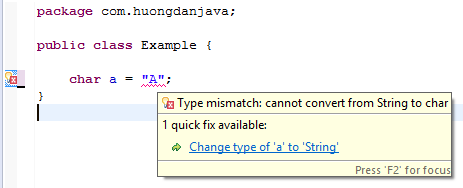A char variable represents 16 bits of Unicode characters, which can represent all characters in all languages, from simple English characters to complex Japanese characters, Korean and also Vietnamese. Its value is expressed in Unicode form between “\u0000” (0) and “\uffff” (65535). And in Java, the char variable is stored with a positive number between 0 and 65535.

In the example above, we declare variable a with the value of A, corresponding to the letter A, which is 65 in the ASCII (American Standard Code for Information Interchange) and thus another way to declare the variable. char in Java that we can use that is:

When running the above code:

The result will be A.

You can refer to the ASCII code table below: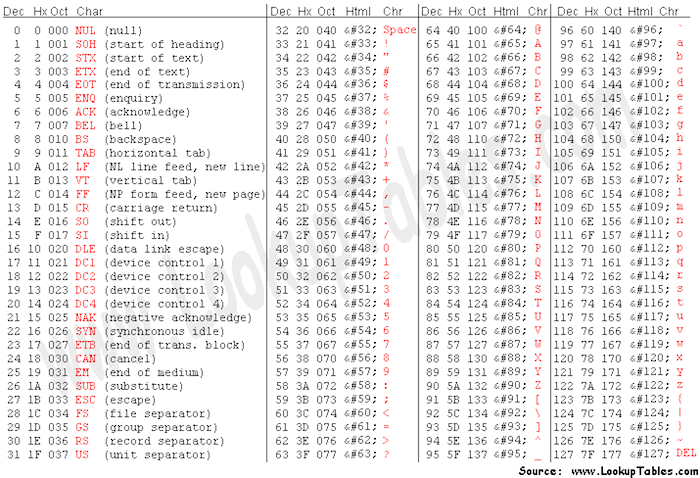As I said above the value of the char variable is a positive number, so if you assign it a negative value, it will have a compile error: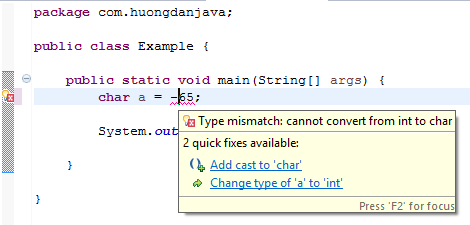But if you declare char variables with negative numbers by pressing the type, it is still ok, no compile error: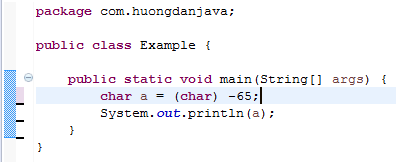But when you run, the result will always be: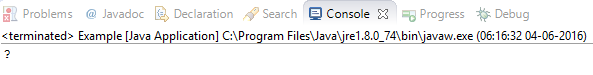### byte

A byte is an 8-bit data type with values ranging from -128 to 127.

The byte variable declaration example is as follows:

### short

short is larger than byte, 16bits, with values greater than bytes from -32.768 to 32.767.

The short variable declaration is as follows:

### int

int is 32 bits in size, ranging from -2,147,483,648 to 2,147,483,647.

Declare the int variable as follows:

### long

Long is a 64-bit data type, its value ranges from -9.223.372.036.854.775.808 to 9.223.372.036.854.775.807.

The variable declaration is as follows:

### Some notes for byte, short, int, and long variables

– When declaring a variable, if you do not assign a value to it, then the default value is 0.

– The default data type of integers is int. Meaning when you declare the following:

the number 1000 is int. But the mechanism of automatic conversion from int to long type of Java allows us to declare such. To specify the number 1000 is the long type, you can add the letter L or l after this number and declare as follows:

In addition, Java allows automatic conversion of variables between primitive types as follows (with both float and double):

• byte to short, int, long, float, or double.
• short to int, long, float, or double.
• char to int, long, float, or double.
• int to long, float, or double.
• long to float or double.
• float to double.

– Java also allows us to represent these variables in binary, decimal, 8th and 16th systems.

• Binary variables are declared starting with 0b or 0B.

For example:

• Decimal is the number we use every day is represented as normal.

For example;

• The 8th system variable is declared starting with 0.

For example:

• The 16th system variable is declared starting with 0x or 0X.

For example:

– In Java 7, when declaring variables with large values, we can use underscores between numbers in your favor so that numbers can be more readable.

For example: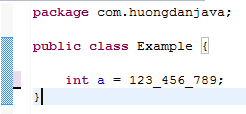You can use underscores to declare variables in Java 7 with the following guidelines:

• You can not declare the underscore at the beginning or at the end of a number.
• You can not put the underscore immediately after 0b, 0B, 0x, 0X in the binary number and number in the 16th system.
• You can put the underscore after the zero in the declaration number in the 8th system.
• You can not put underscores before the letter L or other similar words (the letter L marks the value of the variable in the long type).

This tutorial, I introduced only the first five data types, the next part I will introduce you about float, double and boolean.